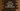# How to use addExact and subtractExact in Java 8## How to use addExact and subtractExact in Java 8 :

In this tutorial, we will learn two new methods added in Java 8 : addExact and subtractExact. These methods are used for addition and subtraction. I will show you how to use these methods with examples.

This method is defined for both integer and long arguments :

``````static int addExact(int a, int b)

static long addExact(long a, long b)``````

So, we can either pass integer or long arguments to this method. It calculates the sum and returns it back. This is a static method and it is defined in java.lang.Math class. Object creation is not required. We can call it directly like Math.addExact(…).

Note that it will throw one exception if the arguments overflow one integer or long.

Let’s take a look at the below example :

``````public class Main {
public static void main(String[] args) {

}
}``````

This program will run without any error. The first println statement will call the addExact(int,int) method and the second one will call the addExact(long,long) method. It will print the sum of the values.

``````300
300680100``````

## subtractExact() :

subtractExact is similar to addExact. The only difference is that it returns the difference of the parameters. This method also has two variants :

``````static int subtractExact(int a, int b)

static long subtractExact(long a, long b)``````

Both are static methods. So, we don’t have to create any object.

It will throw one exception if the result overflows an integer or long.

### Example :

Let’s take a look at the below example :

``````public class Main {
public static void main(String[] args) {
System.out.println(Math.subtractExact(100000,200));

System.out.println(Math.subtractExact(100929292910L, 200445644L));
}
}``````

Both will print the difference of the arguments. Output :

``````99800
100728847266``````